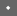7th USENIX Security Symposium, 1998    [Technical Program]

 Pp. 229–242 of the Proceedings# Attack-resistant trust metrics for public key certification

## Abstract

This paper investigates the role of trust metrics in attack-resistant public key certification. We present an analytical framework for understanding the effectiveness of trust metrics in resisting attacks, including a characterization of the space of possible attacks. Within this framework, we establish the theoretical best case for a trust metric. Finally, we present a practical trust metric based on network flow that meets this theoretical bound.

## 1  Introduction

Many public key infrastructures have been proposed and some have been deployed. Almost all, however, suffer from a worrisome problem: a compromise of a single key leads to a successful attack on the entire system. For example, an attacker who gains the root key of a certification hierarchy such as PEM [Ken93] can cause anyone in the entire system to accept an arbitrary forgery (defined to be an incorrect name/key binding, inserted into the system maliciously).

Recent interest in authentication systems centers on systems requiring a certain number of keys (more than one) to be compromised before a forgery is accepted. This work generally focusses on the concept of a trust metric, defined here as a function that computes a trust value from a set of digitally signed certificates. Informally, a good trust metric ensures that there are really multiple independent sources of certification, and rejects (by assigning low trust values) assertions with insufficient certification. The previous work raises many questions, including:

• To which kinds of attack is a trust metric resistant?
• Which trust metric is best?
• How well do these trust metrics work?
This paper answers these questions by analysis of the possible attacks against trust metrics. After introducing a certificate system and its mapping to a graph model (Section 2) and more formally defining the notion of a trust metric (Section 3), we present an analytical framework for quantifying the success of various attacks against trust models (Section 4).

To make the analysis tractable, this paper makes two assumptions. First, we assume that most good name/key bindings are accepted. This assumption is not always valid-for high-security applications, it may be desirable to have a very restricted guest list.'' However, for applications such as key distribution for IP security [Atk95] and widespread secure e-mail, it is a valid assumption. In such applications, if the trust metric often rejects good name/key bindings, then either connections often fail, or else they must be established insecurely. In either case, a trust metric that accepts most good name/key bindings would seem to be a better alternative.

The second assumption is that the name space is opaque, i.e. no information can be gained from the name itself. Most Internet names have some structure, but relationships between names often have little to do with certification relationships, which makes this structure difficult to exploit.

Given this background, the remainder of the paper is devoted to answering the preceding questions, summarized as follows:

• No trust metric can protect against attacks on d keys or more, where d is the minimum number of certifiers on any widely accepted key (Section 5).
• There is an optimal trust metric based on maximum network flow. Such a metric protects against almost any attack on fewer than d keys (Section 6).
• Of previously published trust metrics, the Reiter & Stubblebine [RS97a] trust metric is close to optimal, while the Maurer trust metric [Mau96] is easily attacked (Section 7).

Another contribution of the paper is to distinguish between two different types of attacks on certification systems. The most general form of attack assumes that the attacker is capable of generating arbitrary certificates. This attack corresponds to stealing the secret keys of the victim, and is called a node attack.

However, a far more restricted attack is effective against many of the trust metrics and can be easily mounted without stealing secret keys. It suffices to trick owners of the secret keys into certifying that untrustworthy keys are trustworthy; this attack is called an edge attack. Fortunately, it is possible to design trust metrics to be more resistant to edge attacks than to node attacks.

Figure 1 is a table that briefly summarizes these results. Here, shortest path'' is the trust metric that simply measures the length of the shortest chain from client to target. Maurer'' is a simplified version of the trust metric proposed by Maurer [Mau96]. Reiter & Stubblebine'' is the bounded vertex disjoint path metric as described in [RS97a]. Maxflow'' is the maximum network flow metric optimized for node attacks (Section 6). Maxflow-edge'' is the maximum network flow metric optimized for edge attacks (Section 6.2). In this table, d is the number of certificates issued for each key in the system, and \alpha is a factor indicating the amount of sharing of certification keys, generally in the range of [0.5..1] (see Section 5.4).

 # nodes needed # nodes needed # edges needed to mount to mount to mount successful successful successful metric node attack edge attack edge attack shortest path 1 1 1 Maurer 1 1 d Reiter & Stubblebine d d d Maxflow d d d Maxflow-edge d \alpha d2 \alpha d2 best case d \alpha d2 \alpha d2
Figure 1: A comparison of the trust metrics.

## 2  Certificates and graphs

The input to the trust model is a set of digitally signed certificates, while the evaluation of the trust model is based on a graph. Depending on the details of the certificate formats themselves, a number of different mappings from certificates to graphs are possible. For the purposes of this paper, we use a simple but realistic certificate format and mapping into a graph.

In this example model, there are keys, names, and two types of certificates. A binding certificate is an assertion of the form I believe that subject key k is the key belonging to name n'', signed by an issuer key. A delegation certificate is an assertion of the form I trust certificates signed by subject key k'', again signed by an issuer key. The I'' in these statements refers to the holder of the private key corresponding to the issuer's public key.

This model corresponds fairly closely to the PGP certificate model [PGP95] extended with introducer certificates,'' not present in any current implementation of PGP but proposed as a future extension. It also resembles an X.509 certification system [X509] with opaque names (as opposed to distiguished names) and cross-certification.

This model is somewhat simplistic compared to real-world certification schemes. For example, it includes no time-dependent behavior such as validity periods or revocation. The model does not distinguish different kinds of certificate issuers, which might be users creating their own certificates or trusted third parties. Some of the assumptions regarding the graph structure are more realistic for user-created certificates.

The mapping is as follows: Each key is a node of the graph. In addition, each (key, name) pair is also a node. Each delegation certificate maps to an edge from the node corresponding to the issuer key to the node corresponding to the subject key. Each binding certificate maps to an edge from the node corresponding to the issuer key to the node corresponding to the (key, name) binding. Figure 2 shows an example set of certificates, and Figure 3 shows the corresponding graph.

 certificate type issuer subject delegation key_A key_B delegation key_B key_C delegation key_C key_D binding key_D (key_J, Jack'') delegation key_A key_E delegation key_E key_F binding key_F (key_J, Jack'') delegation key_E key_G delegation key_G key_H delegation key_H key_I binding key_I (key_J, Jack'')

Figure 2: An example set of certificates.Figure 3: The corresponding graph.

Let G be a certificate graph. The nodes of G are either key nodes Vk or target nodes Vt, thus G can be written as (Vk \cup Vt, E). In the example of Figure 3, Vt = {(key_J, Jack")}, and Vk is all the other keys {key_A...key_I}.

## 3  Trust models on graphs

This section defines the notion of a trust model as evaluated on graphs. Given a certificate graph G, a source node s \in Vk, and a target node t \in Vt, the evaluation of the trust model results in a real number, interpreted as the degree to which the source key should trust the target. Formally, the trust metric is represented as a real-valued function M(G, s, t). The trust model is sensitive only to the structure of the graph, not the names. Thus, the trust metric must give the same value for isomorphic graphs. In an automated setting, the source s does not use the real number directly, but simply compares it with a threshold \theta(s) to determine whether the target is trustworthy. Formally, a key s accepts a target t iff M (G, s, t) \geq \theta(s). Little is lost by expressing the trust metric in terms of acceptance rather than real numbers-by performing multiple experiments with different thresholds, it is possible to reconstruct the numbers with high accuracy. Thus, where it is simpler, we use acceptance to characterize trust metrics. To avoid working directly with real values for the \theta function, we often express \theta in percentiles, e.g. \theta (s) is set so that s accepts 95% of all targets.

## 3  Attack models

We consider two different types of attack. In a node attack, the attacker is able to generate any certificate from the attacked key. Thus, in a node attack, the attacker may add arbitrary edges (representing delegation or binding certificates) in the graph. This attack is feasible when the attacker obtains the private keying material, for example by stealing a password.

In an edge attack, by contrast, the attacker is only able to generate a delegation certificate from the attacked key. This attack is feasible when the attacker is able to convince the owner of the attacked key that an untrustworthy subject key is trustworthy.

We also assume that the attacker is capable of removing arbitrary certificates from any key and generating arbitrary certificates from newly created keys under its control. Removing a certificate is realized by performing a denial-of-service attack on the communication of the certificate to the system requesting it.

For each attack scenario, the number of keys attacked is fixed, counting only keys for which certificates are added.

We now formally present the notion of edge and node attacks on graphs. Given an original certificate graph G = (Vk \cup Vt, E), an attack is represented by a new graph G' = (Vk' \cup Vt', E'). All of the nodes and edges in G are presumed to be good.'' The new graph, however, contains at least one new target node x \in Vt' - Vt which represents the forgery. Given a graph G, the graph G' is a possible node attack on the set of keys T iff:This predicate states that G' cannot contain any new edges from nodes in G that are not under attack. Thus, all new edges in G' must come from either a node attack or a new node.

In an edge attack, the edges in G' are further constrained so that no edges from attacked nodes go directly to targets, only to other key nodes.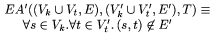The last predicate simply states that attacks must satisfy both the NA and EA' predicates.

A consequence of the EA constraint is that none of the edges from attacked nodes point directly to the target x. Thus, in any path from s to x, the attacked node must be at least distance 2 away from x.

### 4.1  Quantifying the success of an attack

A central contribution of this paper is an analytical framework for quantifying the success of attacks and thus the quality of the trust metric. Trust metrics that make attacks less successful are better.

The success of an attack is most directly measured as the fraction of source keys in Vk accepting the forgery x. Obviously, this fraction depends on the \theta(s) values for the source key. If a source key is tuned to accept very few targets, then it can reject most forgeries as well (in the extreme, accepting no targets is also 100% effective against forgeries). Thus, all measures of success assume fixed target accept rates. For example, it would be reasonable to fix \theta(s) for each source key s so that it accepts 95% of targets. With \theta(s) fixed for each key, it is meaningful to discuss the fraction of source keys that accept a forgery from a specific attack. Formally, the success fraction of an attack G' on a trust metric M is given as: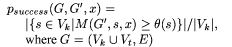For node attacks, it is easier to specify the attack as a set of attacked nodes, rather than an attack graph. The actual graph chosen maximizes the chance of success given the node attack constraint NA.Measuring the success of a particular attack may be useful in some cases, but because the space of individual attacks is so large, it is more useful to characterize trust metrics in terms of their response to classes of attacks. We consider two major classes of attacks: those mounted by randomly choosing nodes to attack, and those mounted by choosing nodes to maximize attack success. In both cases, the attacks are parameterized by the number n of keys attacked.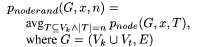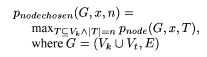It is always the case that pnoderand (G, x, n) \leq pnodechosen (G, x, n) (i.e. a chosen attack is more effective than a random one). The functions pedge, pedgerand, and pedgechosen are all defined analogously, using EA in place of NA.

## 5  Best case analysis

In this section, we address the question: What is the best possible performance of a trust metric? To make this question tractable, we apply two simplifying assumptions. First, we assume the indegree of every node in the graph is a constant d. Second, we assume that most source keys accept most targets in the certificate graph. More formally, \theta(s) of each source s is set so that s accepts the fraction w of all targets. In this analysis, we assume that most clients will want to accept most good targets, so a reasonable value for w would be 99%. Given these assumptions, we prove tight bounds on the best-case performance of any possible graph-based trust metric in resisting attacks.

The assumption of constant indegree d instead of a minimum indegree seems to be necessary to avoid overly centralized graphs, i.e. ones in which most nodes have a single edge to a central node. Such a graph meeting a minimum indegree constraint is easily constructed, but has the obvious weakness that an attack on the central node will cause most keys to accept forgeries.

There are several cases, each of which has a slightly different analysis. The general plan is to describe a feasible attack resulting in a graph G' isomorphic to the original graph G (modulo unreachable nodes), with a forgery x in place of a victim v. No trust metric can distinguish the two graphs, thus clients accept the forgery x with the same success fraction as they accepted the victim v.

The idea of the attack is shown graphically in Figure 4, showing how the original graph of Figure 3} is modified-all edges to the victim, in this case (key_J, Jack''), are removed and replaced with edges to the forgery, here (key_X, Jack''). The nodes attacked (key_D, key_F, and key_I) are highlighted with wedges.Figure 4: An attack.

We consider the following classes of attacks:

• Node attack with a given certificate graph and the attacker chooses the attacked nodes (Section 5.1).
• Node attack with a random certificate graph and the attacked nodes chosen randomly (Section 5.2).
• Node attack with a given certificate graph and the attacker chooses a single attacked node (Section 5.3)
• Edge attack with a given certificate graph and the attacker chooses the attacked nodes (Section 5.4).

In cases where attacks succeed against randomly chosen nodes, the analysis of the chosen node case is not necessary-if the attack works in the former case, it certainly works in the latter. Further, there is a theoretical problem with mounting an attack with randomly chosen attacked nodes against a given certificate graph: the trust metric could just compare the attacked graph against the original graph and reject any certifications if they don't match, an unfair advantage to the trust metric in practice. By showing that the attack works against random graphs, we suggest that it works against most realistic certification graphs.

### 5.1  Node attack; given certificate graph; attacker chooses nodes

In the first case, we assume a fixed certificate graph with the constraint that the indegree of each node is d. The attack is simple. First, the attacker identifies the node v that is accepted by the largest number of keys. Then, the attacker chooses the d predecessors of that node to attack. Finally, the attacker removes v and its predecessor edges (recall that we assume attackers can remove arbitrary edges, see Section 4), and generates new certificates from each of v's predecessors to the forgery node x.

If all keys accept at least the fraction w of targets, then there must be a target v that is accepted by at least the fraction w of the keys (using the pigeonhole principle). Thus, the success fraction is at least w.

The attack works even if the assumption that sources accept w fraction of all good targets is relaxed. It suffices that there is a single target which is widely accepted by a large fraction of clients, which is very likely in any certification system.

### 5.2  Node attack; random certificate graph; attacked nodes chosen randomly

Consider the following procedure for generating a random certificate graph. First choose the number of nodes. Second, for each node n, choose d random predecessors (other than n).

The attack is as follows. Choose a node v at random. Remove v and its predecessor edges. Generate d random edges from the remaining nodes to a new node x.

The resulting graph is clearly a member of the set of graphs that may be generated by the random process. Further, it should be clear that the distribution is identical to that of the original random process for constructing graphs. Therefore, no metric can distinguish between the graphs generated randomly and attacked graphs derived from those generated randomly.

### 5.3  Node attack; given certificate graph; a single node is chosen randomly

For this attack, we assume that the attack is on one node chosen randomly. For certificate graphs with large constant indegree, such an attack cannot be very successful, but for certificate graphs in which a substantial fraction of the nodes have indegree 1 (as is the case with the certificate graph stored on the PGP keyservers [McB96]), the attack can have some success.

The attack only works if the attacked node has a successor v with indegree 1. The attack itself is analogous to those above. Remove v from the graph, generate an edge from the attacked node to x, and generate edges from x to the successors of v. The resulting graph is isomorphic to the original.

Assuming that keys are tuned to accept all good nodes, the success fraction p is equal to the fraction of nodes that have successors of indegree 1 (call this fraction f1). Assuming that keys accept good targets with probability at least w, then the success fraction is at least p = (1 - (1 - w) / (1 - f1)) (this formula is the lower bound of the probability of the conjunction of two events when the events are not guaranteed to be independent).

### 5.4  Edge attack; given certificate graph; attacker chooses nodes

Let pred(v) be the predecessors of v, and let pred2(v) = \cupv' \in pred(v) pred (v'). The attack is as follows: find a node v accepted by a sufficiently large number of keys and that has the smallest pred2 (v), which are the nodes attacked. For each node n in pred(v), generate a new node n'. Remove all the edges from pred2(v) to pred(v), replacing them with edges to the newly created nodes.

The number of nodes that must be attacked is bounded from above by d^2. In practice, there is some \alpha such that there exists a widely accepted node n with \alpha d^2 = |pred2(n)|. In this formulation, \alpha is bounded from above by 1. It is very near one for random graphs, and it is hoped to be fairly high (greater than 0.5, say) for realistic certification graphs.

## 6  Network flow trust metric

This section presents a trust metric based on maximum network flows over the certificate graph. The analysis of this trust metric shows that its performance almost exactly matches the best case bounds presented above.

The trust metric is defined as follows. As before, let s be the source and t be the target. Each node n in the graph is assigned a capacity C(s,t)(n) = max (fs (dist (s, n)), gt (dist (n, t))), where dist (s, t) is the length of the shortest path through the graph from s to t. The trust metric is parameterized by the exact definitions of fs and gt.

In this section, we present fs and gt designed to resist node attacks, but not to do particularly well against edge attacks. Let succ(s) be the set of successors of s. DefineThese values are calculated to result in a network maxflow of 1 for most (s, t) pairs in the certificate graph. These values for fs and gt guarantee that the number of nodes with C(n) > 1/d is no greater than d.

An example is shown in Figure 5, in which each node (except for the source and target nodes) is annotated with its capacity. In this example, d=3, fs(1) = 0.5, fs(2...) = .333, and gt (1...) = 0.333 (actually, the d=3 constraint is only met for the target node-additional edges needed to satisfy d=3 for all nodes are omitted for brevity).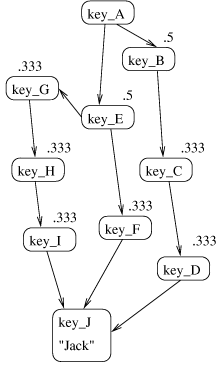Figure 5: Node capacities for network flow trust metric.

This example demonstrates why raising node capacities near the source improves security. If the node capacities were constant, they would need to be set at 0.5 to ensure a maxflow of 1. With these settings, most attacks on two nodes succeed. With capacities raised near the source, the only successful two node attack is on {key_B, key_E}. Considering a random attack, as the graph grows the probability of choosing nodes that are all near any given source key becomes much lower. Even considering a chosen node attack, attacking nodes near one source will not in general be effective against other sources.

In another example, assume a random certificate graph as described in Section 5.2 and the unit capacity maxflow metric. Since roughly half of the nodes in such a graph will have outdegree less than d, the threshold must be set at less than d for approximately half of the keys, implying that half of all source keys accept an attack on d-1 keys.

To summarize, in a well-connected graph (i.e. multiple paths between most nodes), with constant capacities, the trust metric is limited by the minimum of the outdegree of the source and the indegree of the target. With capacities increased near the source, the trust metric is limited only by the indegree of the target.

### 6.1  Analysis: node attacks

The best case analysis shows that a node attack on d nodes is likely to be successful, no matter which trust metric is used. This section shows that, with the network flow trust metric, a node attack on d-1 nodes is unlikely to be successful. Thus, the network flow metric is nearly optimal against node attacks.

We assume an attack where the attacker chooses the nodes to be attacked, as it subsumes the random case (i.e. a trust metric which successfully resists a chosen node attack also resists a randomized attack). We first fix a set S of nodes to attack. Given this set, we analyze the fraction Vk that will accept the forgery. Our goal is to find a tight upper bound on this fraction.

Let us define a node s as susceptible to an attack on S iff there exists a node u in S such that C(s,t)(u) > 1/d. For any set of nodes S where |S| = l there can be no more than ld susceptible nodes, because the indegree d is fixed, and because the set of susceptible nodes is contained in the predecessors of nodes in S. Thus, at least the fraction 1 - ld/|Vk| of all nodes u \in S have C(s,t)(u) = 1/d. We know that S is a cut of G' because the NA predicate ensures that all edges from Vk to the new nodes (Vk' - Vk) originate from the attacked keys. Therefore, the total network flow is bounded by \Sigma_{u \in S}.C(s,t)(u), which in this case is no more than l/d. Thus, for at least 1 - ld/|Vk| of the source nodes, attacks on less than d nodes fail (i.e. for all l < d, pnodechosen (G, x, l) \leq ld/|Vk|).

It should be clear that pnodechosen (G, x, l) falls off very quickly as l decreases. In the case where s has at least d successors, s will not accept any attack on less than d nodes.

### 6.2  Edge attacks

Here are fs and gt tuned to resist edge attacks:Again, we expect there to be a maxmimum flow of 1 for most (s, t) pairs in the graph. For certificate graphs expected to arise in practice, values of \alpha in the range [0.5..1] will lead to a high rate of acceptance. For random graphs, \alpha can be very near unity.

An example is given in Figure 6, in which the immediate successors of s and immediate predecessors of t have capacity 0.333 and all other nodes have capacity .125. The example also shows why a value of 1 for \alpha is not reasonable-in this case, it would cause s to reject t because the predecessors of t share some predecessors. In this example, setting \alpha = 0.888 ensures that s accepts t.Figure 6: Node capacities for metric tuned for edge attacks.

### 6.3  Analysis: edge attacks

The analysis is analogous to that for node attacks, similarly assuming that the attacker chooses the nodes to attack.

Analogous to Section 6.1, let us define a node s as susceptible to an edge attack on S iff there exists a node u for which C(s,t)(u) > 1 / (\alpha d2) and u \not\in pred(t). The number of susceptible nodes is no more than d + d^2, by of the definition of fs. Thus, at least the fraction 1 - k(d + d^2)/|Vk| of all nodes u \in S have C(s,t)(u) = 1 / (\alpha d2).

The total network flow from s to t is bounded by the minimum cut across the nodes, and hence any cut. Consider the cut across nodes S, which in this case is no more than k/(\alpha d^2). Thus, for at least the fraction 1 - k(d + d^2)/|Vk| of all node, edge attacks on less than \alpha d^2 nodes fail.

## 7  Comparison to related work

Our analytical framework for evaluating trust metrics is new but was inspired by the work of Reiter and Stubblebine [RS97a, RS97b]. Their discussion of the bounded vertex disjoint paths trust model gave one criterion for resistance to attack: with a threshold of k independent paths, attacks on k-1 or fewer nodes will never succeed. In [RS97b], Reiter and Stubblebine show a clear example of a trust metric that is not resistant to attack-they demonstrate that in the BBK trust metric [BBK94], an attack on a single node can result in arbitrary manipulation of the trust value. This paper extends their initial work into an analytical framework for comparing trust models in a variety of attack situations.

The general approach of using maximum network flow has been proposed independently by David Johnson, as mentioned in [RS97a]. A different trust metric based on maximum network flow was proposed in [RS97b]. To our knowledge, the idea of increasing the node capacities near the source and target of the query is new. Increasing the capacities near both the source and target has a small effect on node attacks (because the metric is already close to the bound), but does greatly improve resistance to edge attacks-from d to \alpha d^2.

Reiter and Stubblebine [RS97a] propose a trust metric based on counting bounded vertex-disjoint paths. In the absence of a path length bound, this trust metric is equivalent to maximum network flow with unit capacities. The question remains: does the imposition of the path length bound improve the metric? Here, we argue that the answer is no.

Assume that trust metric M1 is maximum flow (without a bound) and a threshold of k1, and that trust metric M2 is bounded vertex disjoint paths and a threshold of k2. For the metrics to be compared directly, source keys must have similar target accept rates. Because the bound causes fewer independent paths to be accepted, k1 > k2. Thus, there is a class of attacks on k2 keys accepted by M2 but not M1. It is important to stress that this analysis depends on the assumption that the metric accepts most good keys. For metrics that accept only a fraction of the good keys, length bounds in metrics may be useful.

An intriguing metric proposed by [Mau96] assigns probabilities to each edge and performs a randomized experiment on whether the target is reachable from the source. Maurer's paper used a different certificate format and mapping than assumed here. Maurer's graphs have two different kinds of edges, but it is possible to consider a simplified form of his model in which there is a single edge. If we assume that the probabilities on the edges are constant across the entire graph, then as the probability goes to zero, the Maurer trust metric becomes consistent with the shortest path trust metric. As the probability goes to one, the Maurer trust metric becomes consistent with a maximum network flow with unit capacities assigned to edges. Appendix A presents proofs of these statements. Such a trust metric is reasonably effective against edge attacks, but succumbs to an attack on a single node. For example, in the graph shown in Figure 7, there are three edge-independent paths from s to t, but such a graph can be compromised by attacking the single node at the bottleneck.Figure 7: A graph with a bottleneck.

## 8  Discussion

We have presented an analytical framework for understanding how a trust metric can be used to resist attacks. Our main assumption is that keys should accept most good targets. We have presented both a best-case theoretical limit on how well trust metrics can perform and a practical trust metric (based on network flows) that meets this limit. Conversely, the fact that the limit is met demonstrates that the attack described in the theoretical analysis (Section 5) is optimal-an attacker should always try to attack the keys nearest the victim node. Under a node attack, the number of nodes that need be attacked is the indegree of the target.

To a first approximation, good trust metrics measure the indegree of the target node. Using a trust metric, then, is only effective if every accepted target has a high indegree. Thus, a trust metric is not particularly helpful on existing certificate graphs such as the PGP key database [McB96], in which about 35% of the keys in the strongly connected subgraph have one predecessor. Each source key has the choice between rejecting a large fraction of the graph or being highly vulnerable to single key forgeries.

We have also distinguished between node attacks (in which the attacker can generate any certificate from a compromised key) and edge attacks (in which the attacker can only generate delegation certificates to an untrustworthy party). We have presented a trust metric that is far more resistant to edge attacks than to node attacks. For realistic values, say d = 10 (every key is certified by at least 10 others) and \alpha = 0.5, an attacker would require 10 keys in a node attack or 50 keys in a edge attack to successfully perpetrate a forgery.

Thus, for an attack-resistant key infrastructure based on trust metrics to be viable, the owner of every key must be willing and able to have a number of people to certify it. Resisting attacks is possible, but increases the cost of certification. Only practical experience with a prototype system can determine whether this tradeoff is worthwhile.

## References

[Atk95]
R.Atkinson. Security Architecture for the Internet Protocol. RFC 1825, IETF (1995).
[BBK94]
T. Beth, M. Borcherding, and B. Klein. Valuation of trust in open netwoks. In D. Gollman, ed., Computer Security -- ESORICS '94 (Lecture Notes in Computer Science 875), pages 3-18, Springer Verlag (1994).
[Ken93]
S. Kent, Internet Privacy Enhanced Mail, Communications of the ACM 36(8), pp.48-60 (1993).
[Mau96]
U. Maurer. Modelling a public-key infrastructure. In E. Bertino, H. Kurth, G. Martella, and E. Montolivo, ed., Computer Security -- ESORICS '96 (Lecture Notes in Computer Science 1146), Springer Verlag (1996).
[McB96]
N. McBurnett. PGP web of trust statistics. https://bcn.boulder.co.us/~neal/pgpstat (1996).
[PGP95]
P. Zimmermann. The Official PGP User's Guide. MIT Press (1995).
[RS97a]
M. Reiter and S. Stubblebine. Path Independence for Authentication in Large-Scale Systems. In Proceedings of the 4th ACM Conference on Computer and Communications Security (1997).
[RS97b]
M. Reiter and S. Stubblebine. Toward Acceptable Metrics of Authentication. In Proceedings of the 1997 IEEE Symposium on Security and Privacy (1997).
[TH92]
A. Tarah and C. Huitema. Associating metrics to certification paths. In Computer Security -- ESORICS '92 (Lecture Notes in Computer Science 648), pages 175-189, Springer Verlag (1992).
[X509]
International Telegraph and Telephone Consultative Committee (CCITT). The Directory -- Authentication Framework, Recommendatation X.509 (1988).

## Appendix A

This appendix presents proofs that the Maurer trust metric becomes consistent with the shortest path and edge-constrained maxflow trust metrics when the edge probability tends to zero or one, respectively.

First, the definition of consistency: Two trust metrics are consistent with each other over a graph G if for all keys, there are no pairs of targets that are ordered inconsistently by the metrics, i.e. M1 and M2 are consistent over G iff:Clearly, the existence of a monotonic function f such that f (M1 (G, s, t)) = M2 (G, s, t) is a sufficient condition that M1 and M2 are consistent with each other.

Consistency is a weaker relationship than equivalence; it is possible for two trust metrics to be consistent even if they differ in granularity. In the extreme case, the constant trust metric is consistent with all other trust metrics. Thus, the Maurer trust metric may be somewhat better'' than its counterparts because of its finer granularity.

Next, we formally define the trust metric. The trust metric described here is actually a simplified version of that presented in [Mau96]. One simplification is that the probability associated with each edge is a constant p; in the original metric, the probability can vary depending on user input, or can be encoded in the certificates themselves. The other major simplification is to use a graph with only one type of edge, a consequence of specifying the subject of a delegation certificate by key, rather than name.

We use MMp to denote the trust metric derived from assigning the probability p to all edges in the graph. We define MMp (G, s, t) to be the probability that, in randomized experiments in which each edge in G is colored black with probability p, there exists a path from s to t consisting entirely of black edges.

We use SP to denote the shortest path metric. SP (G, s, t) is defined as zero minus the shortest path from s to t in graph G, reflecting the fact that shorter paths are to be considered more trustworthy than longer paths.

We use EDP to denote the edge disjoint path metric. EDP (G, s, t) is defined as the number of edge-disjoint paths from s to t in graph G.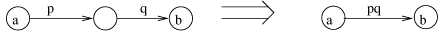Figure 8: Series combination rule for Maurer metric.

### A.1  Proof for p tending to 0

We prove that MMp becomes consistent with SP as p tends to zero. We show this by presenting a monotonic function f that maps MMp to SP, defined as: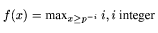We make use of the fact that for monotonic predicates P, j = max_{P(i)} i iff P(i) and \neg P(i+1).

Thus, to show that f(MMp (G, s, t)) = SP (G, s, t), it suffices to show the lower bound MMp (G, s, t) \geq p^{SP (G, s, t)} and the upper bound MMp (G, s, t) < p^{SP (G, s, t) - 1}.

The lower bound is trivial. Let l denote the length of the shortest path from s to t. Consider the subgraph of G containing only a shortest path from s to t. The value of MMp on this subgraph is computed as pl, using the series combination rule (Figure 8). Since the Maurer metric is monotonic (i.e. G' \supseteq G \Rightarrow MMp (G', s, t) \geq MMp (G, s, t)), and SP (G, s, t) = -l, the lower bound follows.

To demonstrate the upper bound, we first compute a breadth-first search of G, for each node n assigning d(n) = the length of the shortest path from s to n. We then calculate, for each path length l, an upper bound u(l) on the probability that at least one node n such that d(n) = l is reachable on an all-black path from s, assuming each edge is colored black with independent probability p.

The calculation is by induction. In the base case, u(0) = 1 trivially.

In the induction step, if no nodes at distance l - 1 are black-reachable, then no nodes at distance l could be black-reachable. Thus, it suffices to compute an upper bound on the conditional probability that at least one node at distance l is black-reachable given that at least one node at distance l - 1 is black-reachable. This conditional probability is no greater than p |E|. Thus, u(l) = p |E| u(l - 1) = (p|E|)l.

The proof is completed by finding a value of p0 such that for all p < p0, u(l) < p^{l - 1}. Solving for equality, we have:

(p |E|)l = p^{l - 1}

p |E|l = 1

p = |E|^{-l}

By choosing p0 to solve this equation for the maximum possible value of l (diam, the diameter of the graph), we satisfy the inequality for all p < p0 and all l \leq diam. Thus, with p0 = |E|^{-diam} the result is proved.Figure 9: Parallel combination rule for Maurer metric.

### A.2  Proof for p tending to 1

We prove that MMp becomes consistent with EDP as p tends to one. We show this by presenting a monotonic function f that maps MMp to EDP, defined as:

f(x) = maxx \geq 1-(1-pdiam)^i : i, i integer

As in the shortest path case, we show that f(MMp(G, s, t)) = EDP (G, s, t) by showing the lower bound MMp(G, s, t) \geq 1-(1-pdiam)^{EDP(G, s, t)} and the upper bound MMp(G, s, t) < 1-(1-pdiam)^{EDP (G, s, t) + 1}.

To show the lower bound, choose the subgraph which contains only k vertex-disjoint paths from s to t. By using the series rule (Figure 8), each path reduces to a single edge of probability pl, where l is the path length. Because no path can be longer than diam edges, the probability on the edge is bounded from below by pdiam. Using the parallel combination rule (Figure 9), these edges reduce to a single edge with probability 1-(1-pdiam)k.

To show the upper bound, consider that because there are only k edge-disjoint paths, there is a set of edges F, |F| = k such that removing those edges blocks all black paths. By computation, the probability of all these edges being removed is (1-p)k. Thus, the probability of an all-black path is bounded from above by 1-(1-p)k. To establish the upper bound, we must show that there exists some p1 such that for all p > p1, 1-(1-p1)k < 1-(1-p1diam)k+1, or equivalently (1-p1)k > (1-p1diam)k+1.

Because pn \geq 1- (1-p)n for all 0 \leq p \leq 1 and all n > 0, this inequality is implied by:

(1-p1)k > ((1-p1)diam)k+1

By simple algebra, this is equvalent to:

p > 1 - 1 / (diamk+1)

Thus, choosing p1 = 1 - 1 / (diamk+1) satisfies the inequality. Because p1 monotonically increases with k, setting it for the highest possible value of k will satisfy the inequality for all s and t.

These two proofs characterize the behavior of the Maurer metric for values of p near 0 and 1, but not for values in between. As might be expected, simulations with the metric over various certificate graphs indicate that its performance is intermediate between these two cases for intermediate values of p.

 This paper was originally published in the Proceedings of the 7th USENIX Security Symposium, January 26-29, 1998, San Antonio, Texas Last changed: 12 April 2002 aw